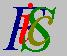Mauro Murzi's pages on Philosophy of Science - Quantum mechanics
AbstractIndex Features of Schrödinger quantum mechanics# 1. Introduction.

The main goal of this article is to provide a mathematical introduction to Schrödinger quantum mechanics suitable for people interested in its philosophical implications.

## Abstract.

A brief explanation of complex functions, including derivatives and partial derivatives, is given. First and second Schrödinger equations are formulated and some of their physical consequences are analysed, particularly the derivation of Bohr energy levels, the forecast of the tunnel effect and an explanation of alpha radioactivity. These examples are chosen in order to show real physical applications of Schrödinger equations. The exposition of Heisenberg indeterminacy principle begins with an analysis of the properties of commutative and non commutative operators, continues with a brief explanation of mean values and ends with some physical applications.

Schrödinger quantum theory is formulated in an axiomatic fashion. No historical analysis is developed to justify the formulation of the two Schrödinger equation: Their only justification derives from their success in explaining physical facts. The philosophical background I use in this article is due to logical positivism and its analysis of the structure of a scientific theory. In this perspective, Schrödinger equations are the theoretical axioms of the theory; the probabilistic interpretation of Schrödinger equations plays the roles of the rules of correspondence, establishing a correlation between real objects and the abstract concepts of the theory; the observational part of the theory describes observation about radioactivity, spectral wavelengths and similar events.Index Features of Schrödinger quantum mechanics